# Mass Weight and Density Quiz 2

#### Quiz Description

Here in this second quiz, we shall go even further into the study of Mass, Weight, and Density. These three are very much related as mass is used in calculations involving weight and density. This quiz is tailored towards the ability to measure the mass of a substance, measure the volume of a substance, calculate the weight of a body, as well as the volume of that body or any given fluid.

Mass is the amount of matter contained in a body. It could also be described to be in direct relationship with the inertia of a body (The reluctance of a body to change its velocity). On the other hand, weight can be defined to be the force that pulls a body towards the earth.

Density on the other hand could be defined as the mass per unit volume of a body or fluid, commonly measured in kilograms per cubic meter (kg/m^3).

This quiz among others is one of the other A-level physics quizzes made available for you. Do you want to fully understand the ideas behind mass, weight, and density? All you need to do is take the quiz and see how much you know and need to know. Good luck as you practice for your exams.

The mass and the volume of a bar made from metal X are measured.

The masses and volumes of four other bars are measured.

Which bar is made from a metal with a density that is double that of X?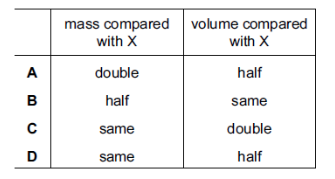Correct
• A
• B
• C
• D

A student collects stones and finds their density.

Which apparatus is needed to measure the mass and the volume of the stones?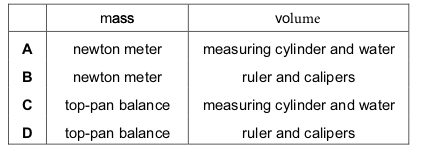Correct
• A
• B
• C
• D

The weight of a stone is found using a newton meter, and its mass is found using a pan balance.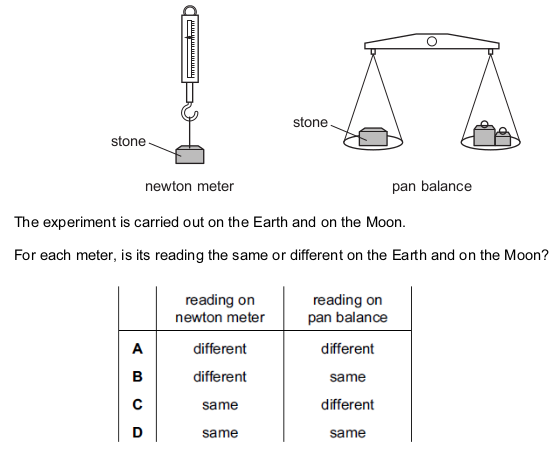Correct
• A
• B
• C
• D

A passenger is sitting in an aeroplane, which takes off and climbs to 10 000 m.

During this time, what happens to the mass and to the weight of the passenger?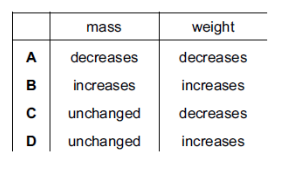Correct
• A
• B
• C
• D

A person of weight 600 N at the bottom of a mountain climbs to the top. The gravitational field strength changes from 10.00 N / kg at the bottom to 9.97 N / kg at the top. His mass is unchanged as he climbs.

What are his mass and his weight at the top of the mountain?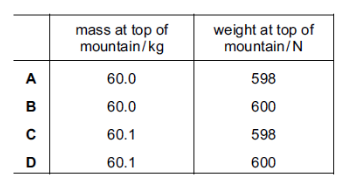Correct
• A
• B
• C
• D

A box has an internal volume of 1000 cm 3 . When a solid object is placed in the closed box, the volume of air in the box is 520 cm 3 .

The density of the object is 8.00 g / cm 3. What is the mass of the object?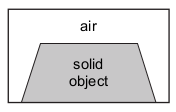Correct
• 60.0 g
• 3840 g
• 4160 g
• 8000 g

A measuring cylinder contains 118 cm 3 of water. When a small object is fully immersed in the water, the reading goes up to 132 cm 3. The object has a mass of 42g.

What is the density of the object?

Correct
• 14 /42 g cm 3
• 42 g /14 cm 3
• 42 g /118 cm 3
• 132 g /42 cm 3

A room measures 4.0 m × 3.0 m × 2.0 m. The density of the air in the room is 1.3 kg / m 3. What is the mass of air in the room?

Correct
• 0.054 kg
• 18 kg
• 24 kg
• 31 kg

A lump of metal has a mass of 210 g. It is lowered into a measuring cylinder containing water.

The level of the water rises from 35 cm 3 to 140 cm 3 .

What is the density of the metal?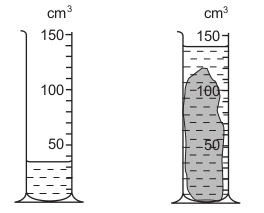Correct
• 0.67 g / cm 3
• 0.67 g / cm 3
• 2.0 g / cm 3
• 6.0 g / cm 3

The mass of a measuring cylinder is measured before and after pouring a liquid into it. What is the density of the liquid?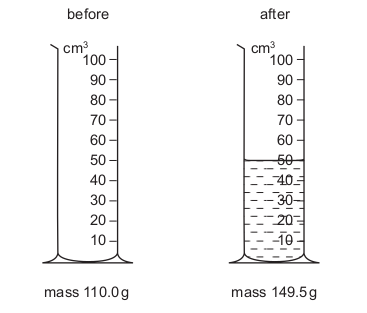Correct
• 0.79 g / cm 3
• 1.3 g / cm 3
• 1.4 g / cm 3
• 2.2 g / cm 3

A student does an experiment to estimate the density of an irregularly-shaped stone. Which items of equipment are needed?

Correct
• a balance and a measuring cylinder containing water
• a balance and a ruler
• a ruler and a measuring cylinder containing water
• only a measuring cylinder containing water

A body of mass 10 kg falling freely in the gravitational field close to the Moon’s surface has an acceleration of 1.6 m / s 2.

What is the gravitational field strength on the Moon?

Correct
• 0 N / kg
• 1.6 N / kg
• 10 N / kg
• 16 N / kg

The inertia of a body is its resistance to changes in motion.

Which property is a measure of the body’s inertia?

Correct
• its density
• its mass
• the height of its sides
• the size of its base

An object of mass 100 g is immersed in water as shown in the diagram. What is the density of the material from which the object is made?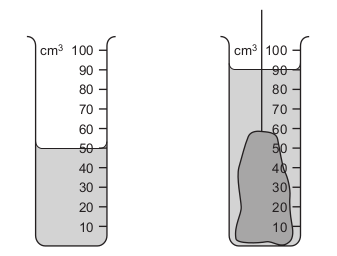Correct
• 0.4 g / cm 3
• 0.9 g / cm 3
• 1.1 g / cm 3
• 2.5 g / cm 3

Three objects are cut from the same sheet of steel. They are different shapes but they all have the same mass. Which object has the greatest density?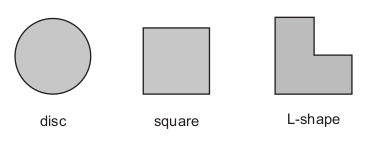Correct
• the disc
• the L-shape
• the square
• they all have the same density

At a point on the surface of the Earth, the gravitational field strength is 9.8 N / kg.

Which pair of values for mass and weight are correct for an object placed at this point?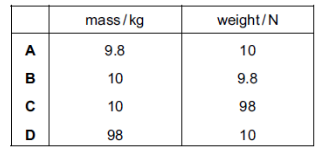Correct
• A
• B
• C
• D

Some students measure the masses and the volumes of different sized samples of a type of wood.

Which graph shows their results?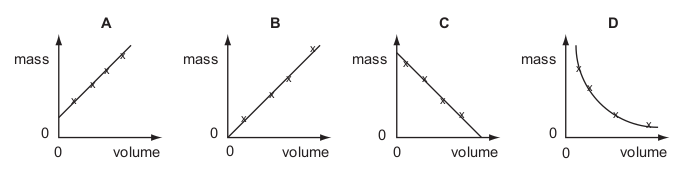Correct
• A
• B
• C
• D

An object that has a mass of 15 kg on the Earth is taken to the Moon.

The gravitational field strength on the Earth is 10 N / kg and on the Moon is 1.6 N / kg.

What are the mass and the weight of the object on the Moon?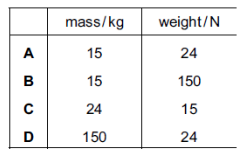Correct
• A
• B
• C
• D

Which relationship defines gravitational field strength?

Correct
• mass x 10
• mass x weight
• mass / weight
• weight / mass

A measuring cylinder containing some water stands on a scale pan. A solid ball is lowered into the water.

The water level rises from the 30 cm 3 mark to the 40 cm 3 mark.

The scale reading increases from 100 g to 180 g.

What is the density of the material of the ball?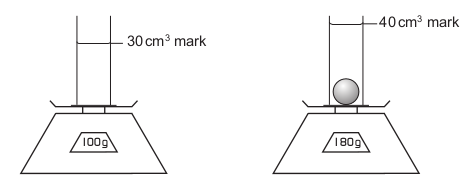Correct
• 2.0 g / cm 3
• 4.5 g / cm 3
• 8.0 g / cm 3
• 18 g / cm 3